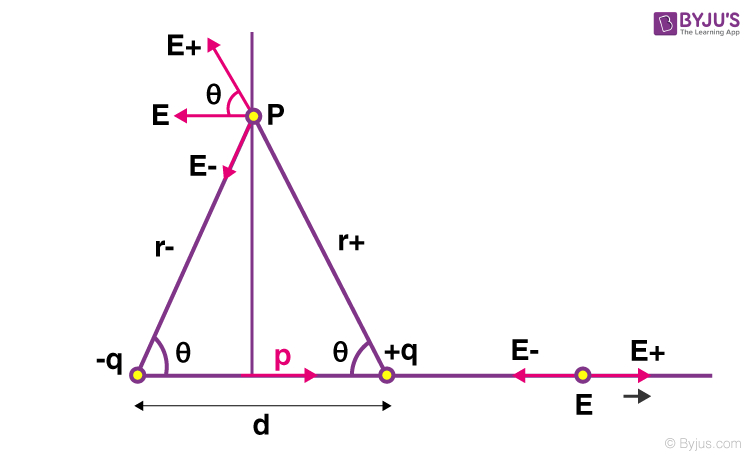# Electric Field of a Dipole

## What Is the Electric Field of a Dipole?

A dipole is a separation of opposite electrical charges and it is quantified by an electric dipole moment. The electric dipole moment associated with two equal charges of opposite polarity separated by a distance, d is defined as the vector quantity having a magnitude equal to the product of the charge and the distance between the charges and having a direction from the negative to the positive charge along the line between the charges.

It is a useful concept in dielectrics and other applications in solid and liquid materials. These applications involve the energy of a dipole and the electric field of a dipole.

## How to Calculate Electric Field of a Dipole?

Consider an electric dipole with charges +q and -q separated by a distance d.We shall for the sake of simplicity only calculate the fields along symmetry axes, i.e. a point P along the perpendicular bisector of the dipole at a distance r from the mid-point of the dipole and a point Q along the axis of the dipole at a distance r from the mid-point of the dipole.

### Along the Perpendicular Bisector (Point P)

The electric fields due to the positive and negative charges (Coulomb’s law):

 $$\begin{array}{l}E_+ = \frac{1}{4πε_0} \frac{q}{r_+^2} \end{array}$$ $$\begin{array}{l} =\frac{1}{4\pi \epsilon _{0}}\frac{q}{(\sqrt{r^2+(\frac{d}{2})^2})^2}\end{array}$$ $$\begin{array}{l} = \frac{1}{4\pi \epsilon _{0}}(\frac{q}{r^2 + (\frac{d}{2})^2})\end{array}$$

Similarly,

$$\begin{array}{l}E_- = \frac{1}{4πε_0}\frac{q}{r_-^2} = \frac{1}{4πε_0} \frac{q}{r^2~+~(\frac{d}{2})^2}\end{array}$$

The vertical components of the electric field cancel out as P is equidistant from both charges.

$$\begin{array}{l} \implies E = E_+~cos~θ~+~E_-~cos~θ\end{array}$$

$$\begin{array}{l} \implies E = \frac{1}{4πε_0} \frac{q}{r^2~+~(\frac{d}{2})^2}~cos~θ~+~ \frac{1}{4πε_0} \frac{q}{r^2~+~(\frac{d}{2})^2}~cos~θ\end{array}$$

$$\begin{array}{l} \implies E = \frac{1}{4πε_0} \frac{2q}{r^2~+~(\frac{d}{2})^2}~cos~θ\end{array}$$

Now,

$$\begin{array}{l} cos~θ= \frac{\frac{d}{2}}{r_+}= \frac{\frac{d}{2}}{r_-}= \frac{\frac{d}{2}}{√{r^2~+~(\frac{d}{2})^2}} \end{array}$$

Substituting this value we get,

$$\begin{array}{l}E=\frac{1}{4πε_0} \frac{2q}{r^2~+~(\frac{d}{2})^2}\frac{\frac{d}{2}}{√{r^2~+~(\frac{d}{2})^2}}=\frac{1}{4πε_0}~ \frac{qd}{(r^2~+~(\frac{d}{2})^2)^{\frac{3}{2}}}\end{array}$$

Dipole moment

$$\begin{array}{l}p = q~×~d\end{array}$$

When r >> d, we can neglect the d/2 term. Thus, we have,

$$\begin{array}{l}E = \frac{1}{4πε_0} \frac{p}{r^2}^{\frac{3}{2}}\end{array}$$

$$\begin{array}{l}\implies E = \frac{1}{4πε_0} \frac{p}{r^3}\end{array}$$

The dipole moment direction is defined as pointing towards the positive charge. Thus, the direction of the electric field is opposite to the dipole moment:

 $$\begin{array}{l}\overrightarrow{E} = -\frac{1}{4πε_0} \frac{\overrightarrow{p}}{r^3}\end{array}$$

### Along Axis of Dipole (Point Q)

The electric fields due to the positive and negative charges are:

 $$\begin{array}{l}E_+ = \frac{1}{4π\epsilon_0} \frac{q}{r_+^2} = \frac{1}{4π\epsilon_0} \frac{q}{(r~-~\frac{d}{2})^2}E_- = \frac{1}{4π\epsilon_0} \frac{q}{r_-^2} = \frac{1}{4π\epsilon_0} \frac{q}{r~+~\frac{d}{2}^2}\end{array}$$

Since the electric fields are along the same line but in opposing directions,

$$\begin{array}{l}E = E_+~-~E_-\end{array}$$

$$\begin{array}{l}E= \frac{1}{4πε_0} \frac{q}{(r~-~\frac{d}{2})^2}~-~\frac{1}{4πε_0}\frac{q}{(r~+~\frac{d}{2})^2}\end{array}$$

$$\begin{array}{l}E = \frac{q}{4πε_0} \left[\frac{1}{(r~-~\frac{d}{2})^2}~-~ \frac{1}{(r~+~\frac{d}{2})^2}\right]\end{array}$$

$$\begin{array}{l}E = \frac{q}{4πε_0} \left[\frac{(r~+~\frac{d}{2})^2~-~(r~-~\frac{d}{2})^2}{(r^2~-~(\frac{d}{2})^2)^2}\right]\end{array}$$

$$\begin{array}{l}E = \frac{q}{4πε_0} \left[\frac{4r \frac{d}{2}}{(r^2~-~(\frac{d}{2})^2)^2}\right]\end{array}$$

$$\begin{array}{l}E = \frac{1}{4πε_0} \left[\frac{2rqd}{(r^2~-~(\frac{d}{2})^2)^2}\right]\end{array}$$

$$\begin{array}{l}E = \frac{1}{4πε_0}~\left[\frac{2rp}{(r^2~-~(\frac{d}{2})^2)^2}\right]\end{array}$$

Factoring r4 from denominator:

$$\begin{array}{l}E = \frac{1}{4πε_0} \frac{1}{r^4} \left[\frac{2pr}{(1~-~(\frac{d}{2r})^2)^2}\right]\end{array}$$

Now if r >> d, we can neglect the (d/2r)2 term becomes very much smaller than 1. Thus, we can neglect this term. The equation becomes:

$$\begin{array}{l} E = \frac{1}{4πε_0} \frac{1}{r^4} \left[\frac{2pr}{1^2}\right]\end{array}$$

$$\begin{array}{l}E = \frac{1}{4πε_0} \frac{2p}{r^3}\end{array}$$

Since in this case the electric field is along the dipole moment, E+ > E,

 $$\begin{array}{l}\overrightarrow{E}= \frac{1}{4πε_0} \frac{2\overrightarrow{p}}{r^3}\end{array}$$

Notice that in both cases the electric field tapers quickly as the inverse of the cube of the distance. Compared to a point charge which only decreases as the inverse of the square of the distance, the dipoles field decreases much faster because it contains both a positive and negative charge. If they were brought to the same point their electric fields would cancel out completely but since they have a small distance separating them, they have a feeble electric field.

## Watch the video and learn about electric field due to dipole## Frequently Asked Questions – FAQs

Q1

### What is an electric dipole?

An electric dipole is defined as a couple of opposite charges q and –q separated by a distance d. By default, the direction of electric dipole in space is always from negative charge -q to positive charge q. The midpoint q and –q is called the centre of the dipole. The simplest example of an electric dipole is a pair of electric charges of two opposite signs and equal magnitude separated by distance.
Q2

### What is the SI unit of the dipole moment?

The SI unit of dipole moment is Coulomb.meter
Q3

### Give an example of an electric dipole.

A pair of electric charges of two opposite signs and equal magnitude separated by a distance.
Q4

### How does an atom behave as a magnetic dipole?

The electrons in an atom revolve around the nucleus in a closed orbit. The orbit around the nucleus is equivalent to a current loop as the electrons are charged particles. The electrons revolve in anticlockwise while the current revolves in the clockwise direction. This movement of electrons creates a south pole and north pole resulting in the atom’s behaviour as a magnetic dipole.
Q5

### What is electric charge?

Electric Charge is the property of subatomic particles that causes it to experience a force when placed in an electric and magnetic field.